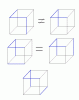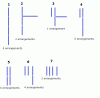BrainDen.com - Brain Teasers

## Question

I have 12 sticks and I have painted 8 of them red and 4 of them blue.

I then glued the sticks together to form the frame of a cube.

So now I'm wondering how many total ways could I have assembled the sticks into cubes that could be distinguished from each other.

Assume I can pick up the cube and move it around any way I like, but I cannot deform or re-assemble it

## Recommended Posts

• 0

Actually, we should split the difference and go with 27.

The variation 4 on the diagram in my previous post should have 8 arrangements.

K-man's zig-zag (5) should have 4, instead of 6 variations.
There are 2 different 3-side zig-zags, and each has 2 (not 3) arrangements with the 4th disjointed side.##### Share on other sites

• 0

Is there a consensus?

Can you construct the possible configurations in groups, using words or a sketch?

My first try to solve this was to take 12 things 4 at a time, then remove the symmetrically equivalent solutions.

But I kept removing too many cases. Next try was to group the edges and distribute 4 blues among the groups.

I'm not convinced yet of my answer.

##### Share on other sites

• 0

Here is how I approached the problem:

1. There is 1 configuration where 4 blue sticks are all on one side of the cube. Let's call it "O" configuration.

2. Now I removed one of them, so 3 remaining blue sticks form a "C" on one side. Since "C" is assymetrical, every position of the 4th blue stick produces a distinct configuration - 8 distinct "C" configurations.

3. The only 1 remaining configuration when all 4 blue sticks are connected is "M" configuration (blue sticks form a letter "M" in isometric projection of the cube).

Next, let's review configurations where 3 blue sticks are connected and the 4th stick is separate excluding any "C" configurations (3+1):

4. There is 1 "Y" configuration where 3 blue sticks connect in one corner (similarly to "M" named after the letter "Y" formed in isometric projection)

5. There are 6 "Z" configurations where 3 blue sticks are connected in a 3D zigzag - it can be left or right oriented and each has 3 possible positions for the 4th blue stick.

Next, configurations where no 3 sticks are connected - "2+2", "2+1+1" and "1+1+1+1":

6. There are 6 "2+2" configurations. To eliminate duplicates I just pick a pair of 2 connected sticks and lock them in one place. Then find all other possible locations for 2 other sticks without being connected to the first 2.

7. There are 4 "2+1+1" configurations. Same idea - lock the 2 connected sticks in place and find possible locations for the remaining 2 without them being connected to the 2 and each other.

8. There are 2 "1+1+1+1" configurations.

So, my total is 1 "O" + 8 "C" + 1 "M" + 1 "Y" + 6 "Z" + 6 "2+2" + 4 "2+1+1" + 2 "1+1+1+1" = 29 configurations.

##### Share on other sites

• 0

Here is how I approached the problem:

1. There is 1 configuration where 4 blue sticks are all on one side of the cube. Let's call it "O" configuration.

2. Now I removed one of them, so 3 remaining blue sticks form a "C" on one side. Since "C" is assymetrical, every position of the 4th blue stick produces a distinct configuration - 8 distinct "C" configurations.

3. The only 1 remaining configuration when all 4 blue sticks are connected is "M" configuration (blue sticks form a letter "M" in isometric projection of the cube).

Next, let's review configurations where 3 blue sticks are connected and the 4th stick is separate excluding any "C" configurations (3+1):

4. There is 1 "Y" configuration where 3 blue sticks connect in one corner (similarly to "M" named after the letter "Y" formed in isometric projection)

5. There are 6 "Z" configurations where 3 blue sticks are connected in a 3D zigzag - it can be left or right oriented and each has 3 possible positions for the 4th blue stick.

Next, configurations where no 3 sticks are connected - "2+2", "2+1+1" and "1+1+1+1":

6. There are 6 "2+2" configurations. To eliminate duplicates I just pick a pair of 2 connected sticks and lock them in one place. Then find all other possible locations for 2 other sticks without being connected to the first 2.

7. There are 4 "2+1+1" configurations. Same idea - lock the 2 connected sticks in place and find possible locations for the remaining 2 without them being connected to the 2 and each other.

8. There are 2 "1+1+1+1" configurations.

So, my total is 1 "O" + 8 "C" + 1 "M" + 1 "Y" + 6 "Z" + 6 "2+2" + 4 "2+1+1" + 2 "1+1+1+1" = 29 configurations.

Very nice approach. Nice categories and elimination of symmetric cases.

In the final 1 + 1 + 1 + 1 case.

Looks like front face has two V [H] edges, and back face has two H [V] edges.

Which [rotation] would be one case, not two?

Maybe I'm missing something.

##### Share on other sites

• 0

Good spacial vision exercise. Without looking at an actual model of a cube, I am not sure I got it right.

Next, let's try it in 4-D.

There are 7 ways in which blue-colored edges of the cube can be conected. And I see the arrangements, which cannot be obtained one from another for each variation as following:So it is 25, unless I missed or duplicated some arrangements.
Edited by Prime
##### Share on other sites

• 0

Here is how I approached the problem:

1. There is 1 configuration where 4 blue sticks are all on one side of the cube. Let's call it "O" configuration.

2. Now I removed one of them, so 3 remaining blue sticks form a "C" on one side. Since "C" is assymetrical, every position of the 4th blue stick produces a distinct configuration - 8 distinct "C" configurations.

3. The only 1 remaining configuration when all 4 blue sticks are connected is "M" configuration (blue sticks form a letter "M" in isometric projection of the cube).

Next, let's review configurations where 3 blue sticks are connected and the 4th stick is separate excluding any "C" configurations (3+1):

4. There is 1 "Y" configuration where 3 blue sticks connect in one corner (similarly to "M" named after the letter "Y" formed in isometric projection)

5. There are 6 "Z" configurations where 3 blue sticks are connected in a 3D zigzag - it can be left or right oriented and each has 3 possible positions for the 4th blue stick.

Next, configurations where no 3 sticks are connected - "2+2", "2+1+1" and "1+1+1+1":

6. There are 6 "2+2" configurations. To eliminate duplicates I just pick a pair of 2 connected sticks and lock them in one place. Then find all other possible locations for 2 other sticks without being connected to the first 2.

7. There are 4 "2+1+1" configurations. Same idea - lock the 2 connected sticks in place and find possible locations for the remaining 2 without them being connected to the 2 and each other.

8. There are 2 "1+1+1+1" configurations.

So, my total is 1 "O" + 8 "C" + 1 "M" + 1 "Y" + 6 "Z" + 6 "2+2" + 4 "2+1+1" + 2 "1+1+1+1" = 29 configurations.

Very nice approach. Nice categories and elimination of symmetric cases.

In the final 1 + 1 + 1 + 1 case.

Looks like front face has two V [H] edges, and back face has two H [V] edges.

Which [rotation] would be one case, not two?

Maybe I'm missing something.

Never mind, I think I see two cases after all. I think that's it.
##### Share on other sites

• 0

Good spacial vision exercise. Without looking at an actual model of a cube, I am not sure I got it right.

Next, let's try it in 4-D.

There are 7 ways in which blue-colored edges of the cube can be conected. And I see the arrangements, which cannot be obtained one from another for each variation as following:colorcube.gif

So it is 25, unless I missed or duplicated some arrangements.

Correction: It is 10 arrangements for the variation 4.

Then the answer is the same as K-man has found.

##### Share on other sites

• 0

Actually, we should split the difference and go with 27.

The variation 4 on the diagram in my previous post should have 8 arrangements.

K-man's zig-zag (5) should have 4, instead of 6 variations.

There are 2 different 3-side zig-zags, and each has 2 (not 3) arrangements with the 4th disjointed side.colorcube2.gif

Good one, Prime! Those two are in fact the same. The drawing certainly helps to see it.

##### Share on other sites

• 0

Someone posed this problem to me, and I tried several ways to count cases and finally gave up.

This prize is a cooperative sharing of k-man and Prime, but I can mark only one post.

So I marked the post that got the final count.

Good job both!

## Join the conversation

You can post now and register later. If you have an account, sign in now to post with your account.×   Pasted as rich text.   Paste as plain text instead

Only 75 emoji are allowed.

×   Your previous content has been restored.   Clear editor

×   You cannot paste images directly. Upload or insert images from URL.